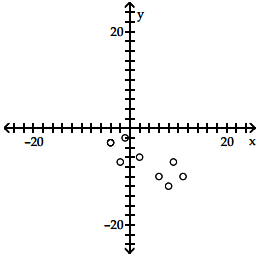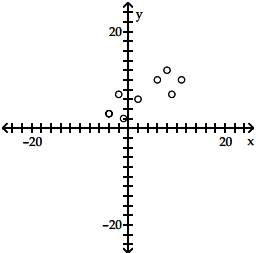Bài sắp đăng
Home | Trắc nghiệm | Kinh tế lượng | 500 câu trắc nghiệm Kinh tế lượng – 9ATổng hợp 500 câu trắc nghiệm + tự luận Kinh tế lượng (Elementary Statistics). Tất cả các câu hỏi trắc nghiệm + tự luận đều có đáp án

# 500 câu trắc nghiệm Kinh tế lượng – 9A

Tổng hợp 500 câu trắc nghiệm + tự luận Kinh tế lượng (Elementary Statistics). Tất cả các câu hỏi trắc nghiệm + tự luận đều có đáp án. Nội dung được khái quát trong 13 phần, mỗi phần gồm 3 bài kiểm tra (A, B, C). Các câu hỏi trắc nghiệm + tự luận bám rất sát chương trình kinh tế lượng, đặc biệt là phần thống kê, rất phù hợp cho các bạn củng cố và mở rộng các kiến thức về Kinh tế lượng. Các câu hỏi trắc nghiệm + tự luận của phần 9A bao gồm:

SHORT ANSWER. Write the word or phrase that best completes each statement or answers the question. Provide an appropriate response.
1) Suppose data are collected concerning the weight of a person in pounds and the number of calories burned in 30 minutes of walking on a treadmill at 3.5 mph. How would the value of the correlation coefficient, r, change if all of the weights were converted to kilograms?

The value of r would remain the same as a change of scale does not affect the value of r.

2) Suppose that statisticians determine that there is a significant positive correlation between the grade earned in the class College Reading Skills and the grade earned in Statistics. Does achieving a high grade in reading cause an individual to earn a high grade in Statistics? Explain your answer with reference to the term lurking variable.

A high grade in the College Reading Skills class does not necessarily cause a high grade in Statistics. These two variables could be related by an underlying relationship. Students who earn high grades in one class tend to earn high grades in other classes perhaps because of a lurking variable — for example, motivation to achieve.

MULTIPLE CHOICE. Choose the one alternative that best completes the statement or answers the question.
Given the linear correlation coefficient r and the sample size n, determine the critical values of r and use your finding to state whether or not the given r represents a significant linear correlation. Use a significance level of 0.05.
3) r = -0.568, n = 25
○ Critical values: r = ±0.487, no significant linear correlation
● Critical values: r = ±0.396, significant linear correlation
○ Critical values: r = ±0.487, significant linear correlation
○ Critical values: r = ±0.396, no significant linear correlation

Find the value of the linear correlation coefficient r.
4) The paired data below consist of the costs of advertising (in thousands of dollars) and the number of products sold (in thousands):

 Cost 9 2 3 4 2 5 9 10 Number 85 52 55 68 67 86 83 73

○ -0.071
● 0.708
○ 0.246
○ 0.235
SHORT ANSWER. Write the word or phrase that best completes each statement or answers the question. Describe the error in the stated conclusion.
5) Given: There is a significant linear correlation between the number of homicides in a town and the number of movie theaters in a town. Conclusion: Building more movie theaters will cause the homicide rate to rise.

Significant correlation does not imply causality. Both variables are affected by a third variable (a lurking variable) , namely the population of the town.

MULTIPLE CHOICE. Choose the one alternative that best completes the statement or answers the
question.
Construct a scatter diagram for the given data.
6)

 x -4 2 8 6 11 9 -2 -1 -4 y 3 6 12 10 10 7 7 2 3